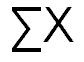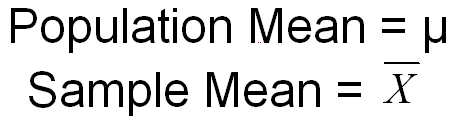﻿ Statistical NotationGraziano & Raulin
Research Methods (9th edition)

## Basics of Statistical Notation

You will be introduced to a large number of formulas in this section on statistical concepts. These formulas use a relatively standardized notation to simplify the description of how a statistic should be computed. This section introduces the logic and basic concepts behind that notation. With each new formula, we will remind you what the notation means, but this section provides a head's up before we get to those formulas and provides a helpful summary in case you forget a notational concept.

### Designating a Variable

• Statistical formulas use algebraic notation, which rely on letters to designate a variable. By convention, if there is just one variable in a formula, the letter X is used to designate the variable. If there is a second variable in the formula, traditionally the letter Y is used to indicate the variable. If there is a third variable, the letter Z is traditionally used. After that, there are no universal traditions, but it is rare to have statistical formulas that involve more than three variables.

• The capital letter N traditionally refers to the total number of participants in a study.

• The single letter in statistical formulas refers to the variable. The individuals scores on that variable can be indicated by subscripts, which are numbers written below the letter to refer to a specific score. For example, X1 refers to the score for the first person on the X variable, and X27 refers to the score for the 27th person on the X variable. Y11 refers to the score on the Y variable for the 11th person.

• If there are several groups of participants, the number of participants in each group is indicated by a lower-case n with a subscript to indicate the group number. For example, n1 refers to the number of participants in the first group.

• Traditionally, the number of groups in a study are referred to by the lower-case letter k, although in complex designs, this tradition is modified. Therefore, nk refers to the number of participants in the kth group, which is the last group.

### Algebraic Rules

• This is a specified order in which functions are to be carried out. The order is:

• The highest priority action should be to raise any variables to a power. For example, to compute 2X2, you would first square the value of X and then multiply by 2.

• The next highest priority action is multiplication or division. For example, to compute 2X +1, you would multiply the value of X by 2 and then add 1.

• The lowest priority action is addition or subtraction.

• You can override any of these priorities by using parentheses. Anything in parentheses should be done before other actions. For example, X + Y2 is computed by squaring Y and adding it to X. In contrast, (X+Y)2 is computed by adding X and Y first and then squaring the sum. In other words, the parentheses in the second equation overrides the normal priority order (raise to a power before adding).

### Summation Notation

• Many statistical formulas involve adding a series of numbers. The notation for adding a series of numbers is the capital Greek letter sigma. The sigma stands for "add up everything that follows." Therefore, if the sigma is followed by the letter X, it means that you should add up all of the X scores.• Parentheses indicate that you should perform the operation in parentheses before you do the summation. For example, the notation below indicates that you should subtract Y from X before you sum the difference.### Standard Notation for Statistics

• A distinction is made between a statistic that is computed on everyone in a population and a the same statistic that is computed on everyone in a sample drawn from the population.

• A statistic computed on everyone in the population is called a population parameter.

• A statistic computed on everyone in a sample is called a sample statistic.

• The population mean is designated by the Greek letter mu, whereas the sample mean is designated by an X with a bar over the top (read X bar). Both are illustrated below.• A similar distinction is made for standard deviation, which is a measure of variability. The population standard deviation in indicated by the lower case Greek letter sigma, whereas the sample standard deviation is indicated by the lower case letter s, as shown below.• The lower case letter r is used to designate a correlation. If there is any doubt about which two variables were used to compute the correlation, the two variables are listed as subscripts. For example, rXY indicates the correlation of X and Y.